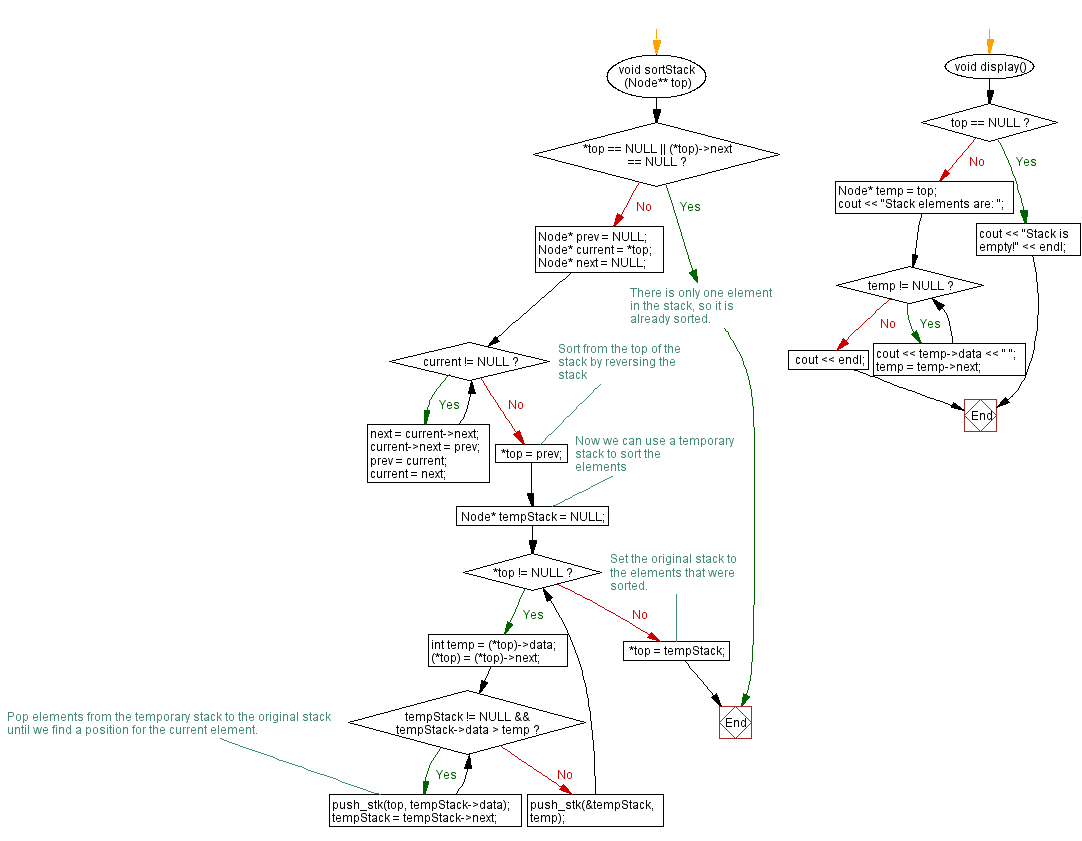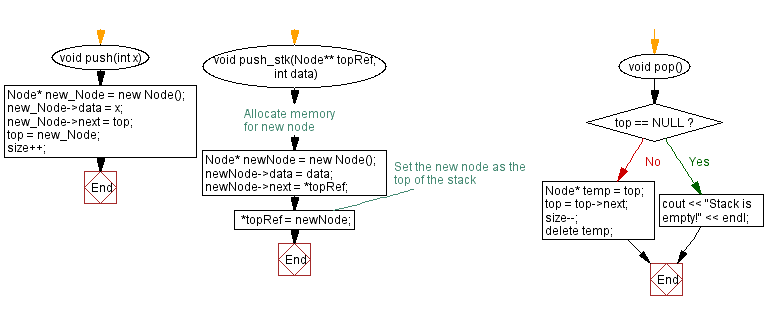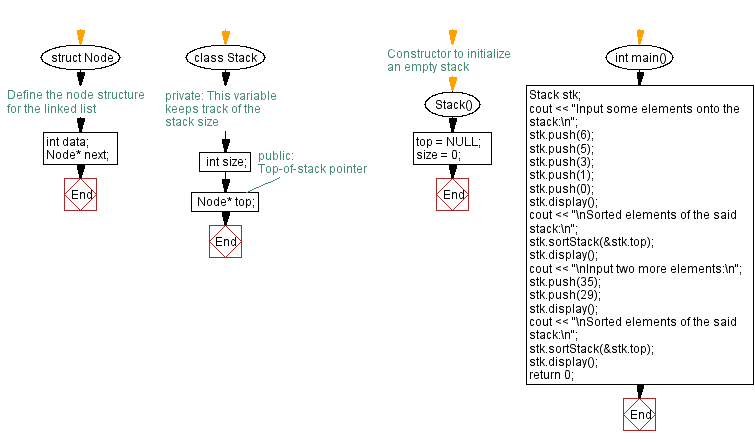﻿ C++ Sort the elements of a stack (using a linked list)

# C++ Stack Exercises: Sort the elements of a stack (using a linked list)

## C++ Stack: Exercise-19 with Solution

Write a C++ program to sort the elements of a stack (using a linked list).

Test Data:
Input some elements onto the stack:
Stack elements are: 0 1 3 5 6
Sorted elements of the said stack:
Stack elements are: 6 5 3 1 0

Sample Solution:

C++ Code:

``````#include <iostream>

using namespace std;

// Define the node structure for the linked list
struct Node {
int data;
Node* next;
};

class Stack {
private:

//This variable keeps track of the stack size
int size;

public:
// Top-of-stack pointer
Node* top;
// Constructor to initialize an empty stack
Stack() {
top = NULL;
size = 0;
}

void push(int x) {
Node* new_Node = new Node();
new_Node->data = x;
new_Node->next = top;
top = new_Node;
size++;
}

void push_stk(Node** topRef, int data) {
// Allocate memory for new node
Node* newNode = new Node();
newNode->data = data;
newNode->next = *topRef;

// Set the new node as the top of the stack
*topRef = newNode;
}
void pop() {
if (top == NULL) {
cout << "Stack is empty!" << endl;
return;
}
Node* temp = top;
top = top->next;
size--;
delete temp;
}

void sortStack(Node** top) {
if (*top == NULL || (*top)->next == NULL) {
// There is only one element in the stack, so it is already sorted.
return;
}

Node* prev = NULL;
Node* current = *top;
Node* next = NULL;

while (current != NULL) {
next = current->next;
current->next = prev;
prev = current;
current = next;
}

// Sort from the top of the stack by reversing the stack
*top = prev;

// Now we can use a temporary stack to sort the elements
Node* tempStack = NULL;
while (*top != NULL) {
int temp = (*top)->data;
(*top) = (*top)->next;

while (tempStack != NULL && tempStack->data > temp) {
// Pop elements from the temporary stack to the original stack
// until we find a position for the current element.
push_stk(top, tempStack->data);
tempStack = tempStack->next;
}

push_stk(&tempStack, temp);
}
// Set the original stack to the elements that were sorted.
*top = tempStack;
}
void display() {
if (top == NULL) {
cout << "Stack is empty!" << endl;
return;
}
Node* temp = top;
cout << "Stack elements are: ";
while (temp != NULL) {
cout << temp->data << " ";
temp = temp->next;
}
cout << endl;
}
};

int main() {
Stack stk;
cout << "Input some elements onto the stack:\n";
stk.push(6);
stk.push(5);
stk.push(3);
stk.push(1);
stk.push(0);
stk.display();
cout << "\nSorted elements of the said stack:\n";
stk.sortStack(&stk.top);
stk.display();
cout << "\nInput two more elements:\n";
stk.push(35);
stk.push(29);
stk.display();
cout << "\nSorted elements of the said stack:\n";
stk.sortStack(&stk.top);
stk.display();
return 0;
}
``````

Sample Output:

```Input some elements onto the stack:
Stack elements are: 0 1 3 5 6

Sorted elements of the said stack:
Stack elements are: 6 5 3 1 0

Input two more elements:
Stack elements are: 29 35 6 5 3 1 0

Sorted elements of the said stack:
Stack elements are: 35 29 6 5 3 1 0
```

Flowchart:CPP Code Editor: